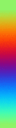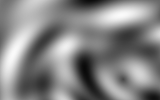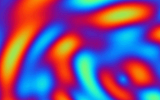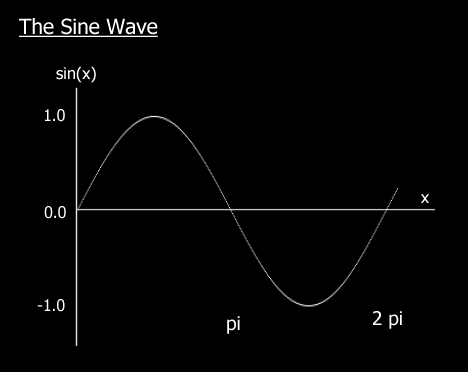×
INTELLIGENT WORK FORUMS
FOR COMPUTER PROFESSIONALS

Are you a
Computer / IT professional?
Join Tek-Tips Forums!
• Talk With Other Members
• Be Notified Of Responses
• Keyword Search
Favorite Forums
• Automated Signatures
• Best Of All, It's Free!

*Tek-Tips's functionality depends on members receiving e-mail. By joining you are opting in to receive e-mail.

#### Posting Guidelines

Promoting, selling, recruiting, coursework and thesis posting is forbidden.

# Plasma

## Plasma

(OP)
I'm trying to get a plasma graphic affect in qbasic. Does ne1 know how to do this?

### RE: Plasma

Check Out My LudaTris Program at my site...

In the Projects or Qbasic section(s)...

Let me know if there are any areas you need help with...

I think I made a Plasma Sub for that program...

Also, for the basics & theory...
Check out the plasma tutorial at
www.flipcode.com/demomaking

Issue 4 is the plasma tutorial...
http://www.flipcode.com/demomaking/issue04.shtml

This is where I learned how to make plasma effects...

And, that is one of the best I have seen so far (until I make one)

Have Fun, Be Young... Code BASIC
-Joshhttp://cubee.topcities.com

### RE: Plasma

(OP)
I sorta get how you do plasma. You use POINT and find the surounding colors and then average them or something like that. but what do you do after it looks plasma like. do u maniplulate the palette  or something?

### RE: Plasma

goto www.qbasic.com > Files > gfx demos.  There are two or three plasma demos there.

### RE: Plasma

Yes, you manipulate the palette using SINs and COSs with the timer to give the palette an appearance of smooth rolling random colors...

From flipcode tutorial...

The c/c++ code is...
for (i=0; i<256; i++)
{
vga->SetColour(
i,
(unsigned char)(32 + 31 * cos( i * PI / 128 + (double)currentTime/74 )),
(unsigned char)(32 + 31 * sin( i * PI / 128 + (double)currentTime/63 )),
(unsigned char)(32 - 31 * cos( i * PI / 128 + (double)currentTime/81 ))
);
};

And the SetColour Function...
void VGA::SetColour( unsigned char i, unsigned char r, unsigned char g, unsigned char b )
{
// output the index of the colour
outp( 0x3c8, i );
// and it's RGB components
outp( 0x3c9, r );
outp( 0x3c9, g );
outp( 0x3c9, b );
};

Which produces this effect on the palette...[Image from www.flipcode.com/demomaking]

To change this...[Image from www.flipcode.com/demomaking]

Into this...[Image from www.flipcode.com/demomaking]

The Basic Translation is:
For i = 0 to 255
' output the index of the colour
OUT &H3C8, i
' and it's RGB components
OUT &H3C9, Int(32 + 31 * Cos( i * PI / 128 + Timer))
OUT &H3C9, Int(32 + 31 * Sin( i * PI / 128 + Timer))
OUT &H3C9, Int(32 - 31 * Cos( i * PI / 128 + Timer))
Next

And don't forget to set PI = 3.1415...

How this Works is...
Sin & Cos produce Waves, and At 0 and 360 degrees they connect, to make the ending the same as the begining...[Image from www.flipcode.com/demomaking]

there are 360 degrees in a circle...
There are 2 * Pi radians in a circle...
So to convert Degrees to radians we always say...
R = D * (Pi * 2) / 360
Or
R = D * Pi / 180

But we replace the degrees with Color, and there are only 256 Colors so, we use...
R = C * (Pi * 2) / 256
Or
R = C * Pi / 128

The Timer is used to offset the wave along the x-axis, to change the colors at a constant speed...

The Cos & Sin functions will return values between -1 and 1
So when you multiply that times 31 you get values between -31 and 31
Then when you Add 32 to that, you get values between 0 and 63...
Which is the range for the Red, Green, & Blue Values for the color palette...

To make the palette shift faster change Timer to Timer * Speed (2 is twice the speed)
Slower: Timer / Speed

If you need more help on this let me know...

For my LudaTris Game, I used this sub...
Sub FLIPPAL(Speed%)
currentTime = Timer * Speed%
For I% = 1 To 255
CR = Int(32 + 31 * Cos(I% * PI / 128 + currentTime / 74))
CG = Int(32 + 31 * Sin(I% * PI / 128 + currentTime / 63))
CB = Int(32 - 31 * Cos(I% * PI / 128 + currentTime / 81))
If GREY% Then
CG = CR: CB = CR
End If
OUT &H3C8, I%
OUT &H3C9, Int(CR)
OUT &H3C9, Int(CG)
OUT &H3C9, Int(CB)
Next
End Sub

Have Fun, Be Young... Code BASIC
-Joshhttp://cubee.topcities.com

### RE: Plasma

(OP)
So this is how it goes.

draw random pixels on the screen
go through each pixel and find the surrounding colors
average those colors
redraw the averages colors on the screen
then manipulate the palette with the timer and triig functions so that its smooth palette changing

I just have 2 question about how you would make different clouds (like bigger or smaller)? And how would you average the points. I know with the point command but what would the formulas be like.

average = POINT(x, y) + POINT(x-1, y)+POINT(x+1,y)+etc...

until you get all the surrounding colors of that one pixel?

thx so much for your help so far.

### RE: Plasma

No...
That is called smoothing / bluring...

but it is faster like this...
Def Seg = &HA000
For Y& = 1 to 198
For X% = 1 to 318
Offset& = Y& * 320 + X%
C% = Peek(Offset&)
CN% = Peek(Offset& - 320)
CS% = Peek(Offset& + 320)
CW% = Peek(Offset& - 1)
CE% = Peek(Offset& + 1)
Poke Offset&, (C% + CN% + CS% + CE% + CW%) \ 5
Next
Next

--------------------------------
To Make it even Faster you can precalculate a division table...

255 (max color) * 5 (pixels to average) = 1275 (total possibilities)
Dim Div5(1275) As Integer
For I = 0 to 1275
Div5(I) = I \ 5
Next

...

Def Seg = &HA000
For Y& = 1 to 198
For X% = 1 to 318
Offset& = Y& * 320 + X%
C% = Peek(Offset&)
C% = C% + Peek(Offset& - 320)
C% = C% + Peek(Offset& + 320)
C% = C% + Peek(Offset& - 1)
C% = C% + Peek(Offset& + 1)
Poke Offset&, Div5(C%)
Next
Next
Def Seg

--------------------------------

As for the actual Plasma Effect...

Plasma 101
By: Josh StriblingThe Random Pixel/Smooth is one method...

A more common way of creating a plasma effect is to use a mathmatical equation to describe the screen...
This is another area you can play around with TrigHere is A snipplet from flipcode (again)...
Function 1 is a sine function of the distance to the origin, and very commonly used for plasma equations:
64 + 63 * (sin(hypot(200 - j, 320 - i) / 16)))

Function 2 is a product of my imagination:
64 + 63 * sin(i / (37 + 15 * cos(j / 74))) * cos(j / (31 + 11 * sin(i / 57))))

You will notice that both functions are contained within the interval [0..127]. Also, when you make up your own functions, watch out for the divide by zero error

And The Basic Translation...
*Note: I is X, J is Y  (I & J are commonly used in place of X & Y, especially when dealing with textures)

Breakdown of function 1...
These formulas Are designed for a 640 x 400 pixel buffer...
Qbasic Can't Handle that, so...
320 - j  -->  160 - X   Returns value between -159 and 160
200 - i  -->  100 - Y   Returns value between -99 and 100

hypot(x, y) returns the hypotenuse of the Right Triangle where:
Y = Opposite
So...
hypot = (X^2 + Y^2)^.5
and in this case...
((160 - X)^2 + (100 - Y)^2)^.5
this will return values between 0 and 187 which is the distance from the center of the screen

You then divide this by the constant (the lower the number the more ripples it will produce, the higher... the less)
since we are using 320 x 200 instead of 640 x 400, we will divide by 8 instead of 16...
You can play with this value to get the desired effect
So then you get a value between 0 and 23.375

Now you get the Sin of that value, which sin will modulate it to 2 * Pi for you... (X Mod 2 * Pi)
Mod returns the remainder of a divison operation... 5 / 2 = 2 r1, so 5 mod 2 = 1
Sin(23.375) is the same as Sin(23.375 Mod 2* Pi)
When the value reaches 2*pi, it will start over creating a continuous wave between -1 and 1...

Now You multiply it by 63 to get a value between -63 and 63

Last but Not least you add 64 to it to get a value between 1 and 127

Which gives us this formula...

64 + 63 * sin(((100 - x)^2 +(160 - y)^2)^.5 / 16))

Now, as you can see this only returns a possible 128 values, and there are 256 color values

So then you take the second function And it its result (which should be 0 to 127) to this to get a color value between 0 and 256

It's that easy...

Breakdown of function 2...
The second formula is a little more complicated, So if you want me to explain it in english also, let me know...
Otherwise, I think I will save some time and give you the scaled and translated formula...

For this one, you can use the original...
64 + 63 * sin(x / (37 + 15 * cos(y / 74))) * cos(y / (31 + 11 * sin(x / 57))))
Or
64 + 63 * sin(x / (19 + 7 * cos(y / 37))) * cos(y / (15 + 5 * sin(x / 29))))
Or you can play with the numbers how ever you like...

In a nut Shell...

Cos(y) & Sin(X) will both produce values between -1 and 1

Sin(x * Cos(Y)) and Cos(Y * Sin(X)) will also = -1 to 1

So, Guess What...
Sin(X * Cos(Y)) * Cos(Y * Sin(X)) still = -1 to 1
Since...
1 * 1 & -1 * -1 both = 1
and
1 * -1 & -1 * 1 both = -1

So you get basically the Same thing As before...
64 + 63 * X
where X = -1 to 1 which creates a value between 1 and 127

So if you use this basic format (64 + 63 * X), you will always get a value between 0 and 127

Now you add the 2 formulas
Color = Formula1 + Formula2

And draw the color to the screen
Pset(X,Y),Color
Or
Def Seg = &hA000
...
Poke Y * 320& + X, Color

The end result will look like this...

'*** Set the screen mode ***
Screen 13

'*** Set The Palette First... Here is a grey scale Palette ***
For I% = 0 To 255
OUT &H3C8, I%
C% = I% \ 4
OUT &H3C9, C%: OUT &H3C9, C%: OUT &H3C9, C%
Next

'*** Draw the plasma (this may take a while) ***
Def Seg = &HA000
For Y% = 0 to 199
For X% = 0 to 319
C1% = 64 + 63 * sin(((100 - x)^2 +(160 - y)^2)^.5 / 16))
C2% = 64 + 63 * sin(x / (19 + 7 * cos(y / 37))) * cos(y / (15 + 5 * sin(x / 29))))
Poke CLng(Y% * 320& + X%), C1% + C2%
Next
Next

'*** Then loop through the Palette Cycle until a key is pressed***
K% = Inp(96)
Speed% = 2
Do
currentTime = Timer * Speed%
For I% = 1 To 255
OUT &H3C8, I%
OUT &H3C9, Int(32 + 31 * Cos(I% * PI / 128 + currentTime / 74))
OUT &H3C9, Int(32 + 31 * Sin(I% * PI / 128 + currentTime / 63))
OUT &H3C9, Int(32 - 31 * Cos(I% * PI / 128 + currentTime / 81))
Next
Loop until Inp(96) <> K%

'*** Restore Palette and End ***
Palette
End

I hope this helps (my fingers are almost numb)
Have Fun Playing with Plasma (it's safer than fire),-Josh

Have Fun, Be Young... Code BASIC
-Joshhttp://cubee.topcities.com

### RE: Plasma

(OP)
........man you typed out all that. no wonder your fingers are numb. Well seriosly I can't thank you enough for helpin me out. Those formulas are confusing, but I think I understand them. The only thing I'm pretty much confused about is using the TIMER in the equation to slow it down. I get how the TIMER changes at a constant rate but doesn't it return a really big number (if its not near after midnight). So how does making the TIMER # bigger make it faster. But at least I understand the SIN and COS better now. Thx a lot#### Red Flag This Post

Please let us know here why this post is inappropriate. Reasons such as off-topic, duplicates, flames, illegal, vulgar, or students posting their homework.

#### Red Flag Submitted

Thank you for helping keep Tek-Tips Forums free from inappropriate posts.
The Tek-Tips staff will check this out and take appropriate action.

Close Box

# Join Tek-Tips® Today!

Join your peers on the Internet's largest technical computer professional community.
It's easy to join and it's free.

Here's Why Members Love Tek-Tips Forums:

•Talk To Other Members
• Notification Of Responses To Questions
• Favorite Forums One Click Access
• Keyword Search Of All Posts, And More...

Register now while it's still free!